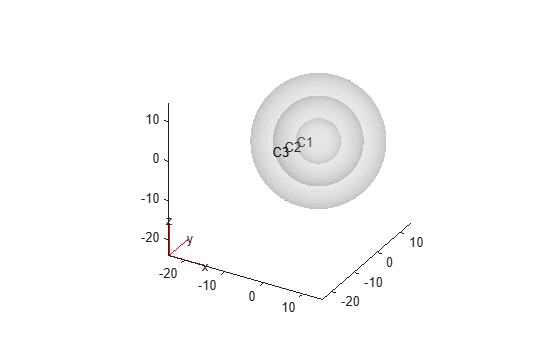# multisphere

Create geometry formed by several spherical cells

## Syntax

``gm = multisphere(R)``
``gm = multisphere(R,'Void',eci)``

## Description

example

````gm = multisphere(R)` creates a geometry by combining several spherical cells.When creating each sphere, `multisphere` uses the following coordinate system.```

example

````gm = multisphere(R,'Void',eci)` creates a multi-sphere geometry with empty cells.```

## Examples

collapse all

Create a geometry that consists of three nested spheres and include this geometry in a PDE model.

Create the geometry by using the `multisphere` function. The resulting geometry consists of three cells.

`gm = multisphere([5 10 15])`
```gm = DiscreteGeometry with properties: NumCells: 3 NumFaces: 3 NumEdges: 0 NumVertices: 0 Vertices: [] ```

Create a PDE model.

`model = createpde`
```model = PDEModel with properties: PDESystemSize: 1 IsTimeDependent: 0 Geometry: [] EquationCoefficients: [] BoundaryConditions: [] InitialConditions: [] Mesh: [] SolverOptions: [1x1 pde.PDESolverOptions] ```

Include the geometry in the model.

`model.Geometry = gm`
```model = PDEModel with properties: PDESystemSize: 1 IsTimeDependent: 0 Geometry: [1x1 DiscreteGeometry] EquationCoefficients: [] BoundaryConditions: [] InitialConditions: [] Mesh: [] SolverOptions: [1x1 pde.PDESolverOptions] ```

Plot the geometry.

`pdegplot(model,'CellLabels','on','FaceAlpha',0.2)`Create a geometry that consists of a single sphere and include this geometry in a PDE model.

Use the `multisphere` function to create a single sphere. The resulting geometry consists of one cell.

`gm = multisphere(5)`
```gm = DiscreteGeometry with properties: NumCells: 1 NumFaces: 1 NumEdges: 0 NumVertices: 0 Vertices: [] ```

Create a PDE model.

`model = createpde`
```model = PDEModel with properties: PDESystemSize: 1 IsTimeDependent: 0 Geometry: [] EquationCoefficients: [] BoundaryConditions: [] InitialConditions: [] Mesh: [] SolverOptions: [1x1 pde.PDESolverOptions] ```

Include the geometry in the model.

`model.Geometry = gm`
```model = PDEModel with properties: PDESystemSize: 1 IsTimeDependent: 0 Geometry: [1x1 DiscreteGeometry] EquationCoefficients: [] BoundaryConditions: [] InitialConditions: [] Mesh: [] SolverOptions: [1x1 pde.PDESolverOptions] ```

Plot the geometry.

`pdegplot(model,'CellLabels','on')`Create a hollow sphere and include it as a geometry in a PDE model.

Create a hollow sphere by using the `multisphere` function with the `Void` argument. The resulting geometry consists of one cell.

`gm = multisphere([9 10],'Void',[true,false])`
```gm = DiscreteGeometry with properties: NumCells: 1 NumFaces: 2 NumEdges: 0 NumVertices: 0 Vertices: [] ```

Create a PDE model.

`model = createpde`
```model = PDEModel with properties: PDESystemSize: 1 IsTimeDependent: 0 Geometry: [] EquationCoefficients: [] BoundaryConditions: [] InitialConditions: [] Mesh: [] SolverOptions: [1x1 pde.PDESolverOptions] ```

Include the geometry in the model.

`model.Geometry = gm`
```model = PDEModel with properties: PDESystemSize: 1 IsTimeDependent: 0 Geometry: [1x1 DiscreteGeometry] EquationCoefficients: [] BoundaryConditions: [] InitialConditions: [] Mesh: [] SolverOptions: [1x1 pde.PDESolverOptions] ```

## Input Arguments

collapse all

Cell radius, specified as a positive real number or a vector of positive real numbers. If `R` is a vector, then `R(i)` specifies the radius of the `i`th cell.

Example: `gm = multisphere([1,2,3])`

Empty cell indicator, specified as a vector of logical `true` and `false` values. This vector must have the same length as the radius vector `R`.

The value `true` corresponds to an empty cell. By default, `multisphere` assumes that all cells are not empty.

Example: ```gm = multisphere([1,2,3],'Void',[false,true,false])```

## Output Arguments

collapse all

Geometry object, returned as a DiscreteGeometry Properties object.